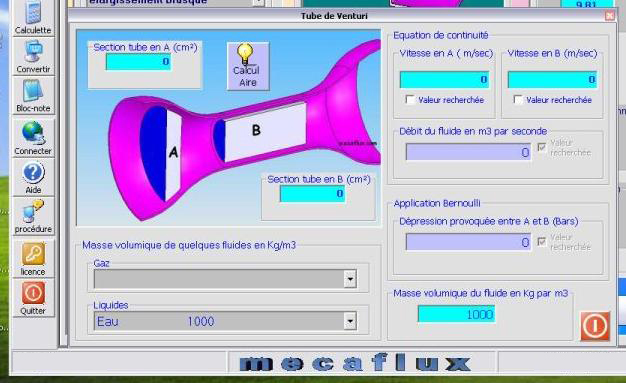Bernoulli Théorèm

The sum of the pressures and mechanical energy per unit volume, is constant along the flow tube.

or: FORMULA Bernoulli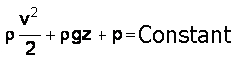Pressure Kinetics + Pressure of weight + Pressure energy = constant

r is the density in Kg/m3.

V is fluid velocity in m/s.

g is the gravity 9.81 m/s².

Z is the vertical drop of the pipe in meters.

P is the static pressure in pascals.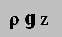is the pressure of weight or potential energy.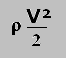is the kinetic energy or kinetic pressure.

The Bernoulli equation can be considered as a principle of conservation of energy, suitable for moving fluids.The behavior usually called "Venturi effect" or "Bernoulli effect" is the reduction of fluid pressure in areas where the flow velocity is increased. This decrease in pressure in a narrowing of the duct may seem contradictory, unless you consider the pressure as an energy density. Passage through the narrowing, the fluid velocity, so its kinetic energy should be increased at the expense of pressure energy.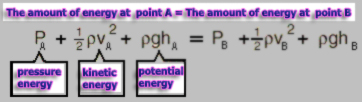Assuming that the flow is laminar, the major pressure losses of the duct are negligible, and that the speed is the average speed:

The Tools menu mecaflux software contains an application for, use the Bernoulli to determine the operating conditions of a system, according to the parameters of variation of speed, altitude and pressure between two points of a fluid stream.. like a venturi system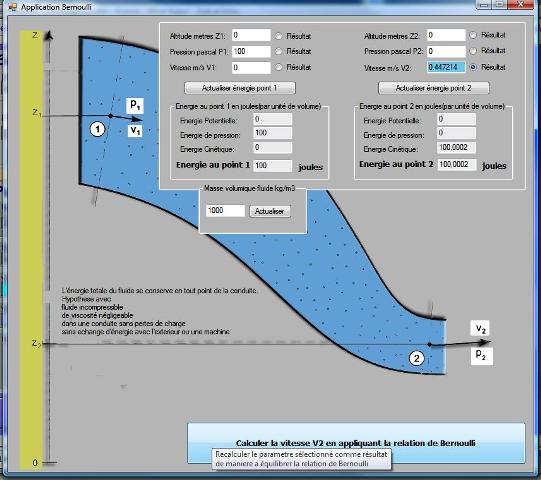the tool venturi of the mecaflux software is also an application of the Bernoulli equation: